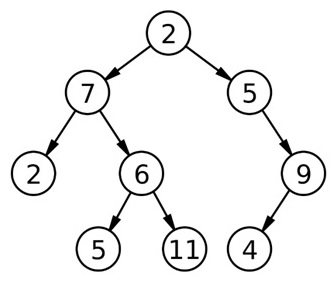# Level order traversal in spiral form

In this article, we are going to see how to print level order traversal in spiral form of a binary tree? This problem has been featured in coding round of Amazon.
Submitted by Radib Kar, on April 07, 2019

Problem statement:

Write a program to print Level Order Traversal in spiral form of a binary tree.

Example:```For the above tree:
Basic level order traversal:
2
7 5
2 6 9
5 11 4
Level order traversal in spiral form:
2
7 5 (left to right)
9 6 2 (right to left)
5 11 4 (again left to right)
```

Solution:

The solution will, of course, surround basic level order traversal. The spiral order means - It will go from left to right for one level, then right to left for next level and again left to right for the next one and so on.

We need to modify our basic level order traversal.

We can do the flipping of direction (left → right then right → left so on ...) by keeping a flag variable which will be updated at end of each level.

Pre-requisite: Root to tree

```1.  Declare flag as 1(true);
2.  Declare a queue q to store pointer to nodes(node*);
3.  Declare a stack s which helps us for flipping.
4.  Print the root as we are not going to bother about root level;
5.  IF(root->left) //left child exists
ENQUEUE(q, root->left);
END IF
IF(root->right) //right child exists
ENQUEUE(q, root->right);
END IF
IF root has no child
RETURN BACK //nothing to print more
ELSE
q.push(NULL); //to indicate end of 1st level

6.  //Here goes the modified level order traversal
When flag=1 its left-to right
flag=0 its right to left
while (q is not empty){
temp=DEQUEUE(q);
IF(temp==NULL) //end of last traversed level
IF (q is not empty)
ENQUEUE (q, NULL);
END IF
IF (flag==0)
Pop and print data from stack until stack is empty
END IF
flag=1-flag; //flip flag for next level 1 to 0 or 0 to 1
ELSE
IF(flag == 1)
Print temp->data; //left to right printing
ELSE
Push temp->data to stack s; //this makes right to left
//printing as rightmost node will be at the top of stack
END IF-ELSE
// basic level order traversal (direction left to right)
IF(root->left) //left child exists
ENQUEUE(q, root->left);
END IF
IF (root->right) //right child exists
ENQUEUE(q, root->right);
END IF
END IF-ELSE (outer one)
END WHILE loop
```

Example with Explanation:

```    For the above tree root is being printed

first without any constraint
2

For the first level flag is 1

Thus it prints immediately while accessing temp node
7 5 (since basic traversal direction is always left to right)

At the end of level flag flips to 0

So while traversing instead of printing nodes at once,
nodes get stored in stack

At the end of level all the nodes data being popped and printed.
In stack
9(top)
6
2

Thus printing
9 6 2 (right to left)

Flag again flipped to 1

Basic left to right printing
5 11 4
So the output is in spiral order
```

C++ implementation:

```#include <bits/stdc++.h>
using namespace std;

// tree node is defined
class Node{
public:
int data;
Node *left;
Node *right;
};

// creating new node
Node* newnode(int data)
{
Node* node = (Node*)malloc(sizeof(Node));
node->data = data;
node->left = NULL;
node->right = NULL;
return(node);
}

void printSpiral(Node *root)
{
Node* temp;
int flag=1;
queue<Node*> q;
stack<int> s;

cout<<root->data<<"\n";
if(root->left)
q.push(root->left);
if(root->right)
q.push(root->right);
if(!root->left && !root->right)
return;

q.push(NULL);

while(!q.empty()){
temp=q.front();
q.pop();
if(temp==NULL){
if(!q.empty())
q.push(NULL);
if(flag==0){
while(!s.empty()){
cout<<s.top()<<" ";
s.pop();
}
}
flag=1-flag;
cout<<endl;
}
else{
if(flag){
cout<<temp->data<<" ";
}
else{
s.push(temp->data);
}
if(temp->left)
q.push(temp->left);
if(temp->right)
q.push(temp->right);
}
}
}

int main() {
//**same tree is builted as shown in example**
Node *root=newnode(2);
root->left= newnode(7);
root->right= newnode(5);
root->right->right=newnode(9);
root->right->right->left=newnode(4);
root->left->left=newnode(2);
root->left->right=newnode(6);
root->left->right->left=newnode(5);
root->left->right->right=newnode(11);

cout<<"Level Order traversal in spiral form of ";
cout<<"the binary tree is :"<<endl;
printSpiral(root);

return 0;
}
```

Output

```Level Order traversal in spiral form of the binary tree is :
2
7 5
9 6 2
5 11 4
```